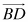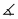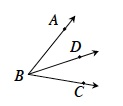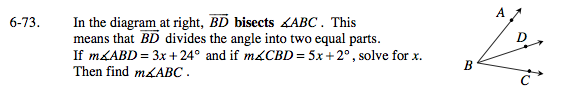Home > GC > Chapter 6 > Lesson 6.2.4 > Problem6-73

6-73.

In the diagram below,bisectsABC . This means thatdivides the angle into two equal parts. If mABD = 3x + 24° and if mCBD = 5x + 2°, solve for x. Then find mABC. Homework Help ✎$\text{If }\overline{BD} \text{ divides }\angle ABC\text{ into two equal parts, what can you do with the equations for their measures?}$

3x + 24° = 5x + 2°

Once you have solved for x, substitute it back into the expressions of each angle.
How can you use those measures to find the measure of ∠ABC?